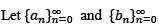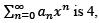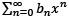Courses

#be sequences of positive real numbers such that nan < bn < n2an forall n > 2. If the radius of convergence of the power seriesthen the power seriesa)converges for all x with |x| > 2b)converges for all x with  |x| < 2c)does not converge for any x with  |x| > 2d)does not converge for any x with  |x| < 2Correct answer is option 'B'. Can you explain this answer? Related Test: Math - 2019 Past Year Paper

## IIT JAM QuestionDronacharya Institute Jan 19, 2021

This discussion on be sequences of positive real numbers such that nan < bn < n2an forall n > 2. If the radius of convergence of the power series then the power seriesa)converges for all x with |x| > 2b)converges for all x with |x| < 2c)does not converge for any x with |x| > 2d)does not converge for any x with |x| < 2Correct answer is option 'B'. Can you explain this answer? is done on EduRev Study Group by IIT JAM Students. The Questions and Answers of be sequences of positive real numbers such that nan < bn < n2an forall n > 2. If the radius of convergence of the power series then the power seriesa)converges for all x with |x| > 2b)converges for all x with |x| < 2c)does not converge for any x with |x| > 2d)does not converge for any x with |x| < 2Correct answer is option 'B'. Can you explain this answer? are solved by group of students and teacher of IIT JAM, which is also the largest student community of IIT JAM. If the answer is not available please wait for a while and a community member will probably answer this soon. You can study other questions, MCQs, videos and tests for IIT JAM on EduRev and even discuss your questions like be sequences of positive real numbers such that nan < bn < n2an forall n > 2. If the radius of convergence of the power series then the power seriesa)converges for all x with |x| > 2b)converges for all x with |x| < 2c)does not converge for any x with |x| > 2d)does not converge for any x with |x| < 2Correct answer is option 'B'. Can you explain this answer? over here on EduRev! Apart from being the largest IIT JAM community, EduRev has the largest solved Question bank for IIT JAM.

### Related Content

This discussion on be sequences of positive real numbers such that nan < bn < n2an forall n > 2. If the radius of convergence of the power series then the power seriesa)converges for all x with |x| > 2b)converges for all x with |x| < 2c)does not converge for any x with |x| > 2d)does not converge for any x with |x| < 2Correct answer is option 'B'. Can you explain this answer? is done on EduRev Study Group by IIT JAM Students. The Questions and Answers of be sequences of positive real numbers such that nan < bn < n2an forall n > 2. If the radius of convergence of the power series then the power seriesa)converges for all x with |x| > 2b)converges for all x with |x| < 2c)does not converge for any x with |x| > 2d)does not converge for any x with |x| < 2Correct answer is option 'B'. Can you explain this answer? are solved by group of students and teacher of IIT JAM, which is also the largest student community of IIT JAM. If the answer is not available please wait for a while and a community member will probably answer this soon. You can study other questions, MCQs, videos and tests for IIT JAM on EduRev and even discuss your questions like be sequences of positive real numbers such that nan < bn < n2an forall n > 2. If the radius of convergence of the power series then the power seriesa)converges for all x with |x| > 2b)converges for all x with |x| < 2c)does not converge for any x with |x| > 2d)does not converge for any x with |x| < 2Correct answer is option 'B'. Can you explain this answer? over here on EduRev! Apart from being the largest IIT JAM community, EduRev has the largest solved Question bank for IIT JAM.